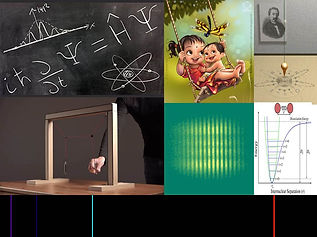top of page# Physics 1 (PH 103, For First year B.Tech)

Course contents                                     3-1-0-8         Pre-requisites: nil

Orthogonal coordinate systems and frames of reference, conservative and non-conservative forces , work-energy theorem, potential energy and concept of equilibrium; Rotation about fixed axis, translational-rotational motion, vector nature of angular velocity, rigid body rotation and its applications, Euler's equations; Gyroscopic motion and its application; Accelerated frame of reference, centrifugal and Coriolis forces.

Harmonic oscillator, damped and forced oscillations, resonance, coupled oscillations, small oscillation, normal modes, longitudinal and transverse waves, wave equation, plane waves, phase velocity, superposition wave packets and group velocity, two and three dimensional waves.

Failure of classical concepts, Black body radiation, photo-electric effect, Compton effect, Davison and Germer's experiment, Frank-Hertz experiment, Bohr's theory, Sommerfeld's model, correspondence principle, Planck hypothesis, De Broglie's hypothesis, Hilbert space, observables, Dirac notation, principle of superposition, wave packets, phase and group velocities, probability & continuity equation, eigenvalues and eigenfunctions, orthonormality, expectation values, uncertainty principle, postulates of QM, Schrodinger equation & its applications to 1D potentials, field quantization, periodic potential wells: Kronig Penny model and origin of band gap.

Textbooks:

• D. Kleppner and R. J. Kolenkow, An introduction to Mechanics, Tata McGraw-Hill, New Delhi, 2000.

• David Morin, Introduction to Classical Mechanics, Cambridge University Press, NY, 2007.

• Frank S. Crawford, Berkeley Physics Course Vol 3: Waves and Oscillations, McGraw Hill, 1966.

• Eyvind H. Wichmann, Berkeley Physics Course Vol 4: Quantum physics, McGraw Hill, 1971.

## ​

## ​

Transformation of vectors from Cartesian to

polar co-ordinates

Velocity and acceleration

## ​TUTORIAL 1 (9/August/2019)

## ​#### LECTURE 3  (13/August/2019)

Cylidrical polar co-ordinate system

• Transformation of vectors

• Infinitesimal line, area and volume element## LECTURE 4  (16/August/2019)

Spherical polar co-ordinate system

• Transformation of vectors

• Infinitesimal line, area and volume element

bottom of page